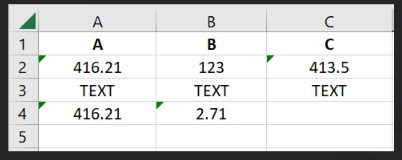# How to Find the difference between two cell values and compare it with other cell value in Excel?

Hi Community,I would like to know what would be the data type for A,B,C columns. The values are dynamic. It can be String, number, decimals, etc

So, I need to find the difference between A and B and verify if it’s matching with C or not except last column . (Sometimes A value can be bigger, and sometimes B value can be bigger)

Approach I Tried is throwing error at Get row item activity. If it’s output variable is String type.
If I change it to Double, it is throwing TEXT is not a valid value for Double.
If I change to Generic Value, In If condition(A isNumeric = true AND B isNmeric = True) , It’s throwing error.

Any Guidance would be appreciatedThanks in AdvanceYou can use the Generic Value data type for all three columns. Try the following query in Invoke Code activity:

``````Dim aValue As Double = 0
Dim bValue As Double = 0
Dim cValue As String

Dim isANumeric As Boolean = IsNumeric(row("A").ToString())
Dim isBNumeric As Boolean = IsNumeric(row("B").ToString())

If isANumeric AndAlso isBNumeric Then

Double.TryParse(row("A").ToString(), aValue)
Double.TryParse(row("B").ToString(), bValue)
Dim difference As Double = Math.Abs(aValue - bValue)

cValue = row("C").ToString()
If Not String.IsNullOrEmpty(cValue) AndAlso IsNumeric(cValue) AndAlso difference = Convert.ToDouble(cValue) Then
Else
[YOUR CODE FOR NON MATCHING BLOCK]
Else
[VALUES ARE NOT DOUBLE BLOCK]

End If

Catch ex As Exception
[EXCEPTION HANDLING BLOCK]
End Try
``````

Hope this helps,
Best Regards.

Hi,